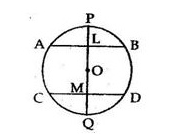# If a diameter of a circle bisects each of the two chords of a circle then prove that the chords are parallel.

Question:

If a diameter of a circle bisects each of the two chords of a circle then prove that the chords are parallel.

Solution:

Given: AB and CD are two chords of a circle with centre O. Diameter POQ bisects them at points L and M.
To prove: AB || CD
Proof: AB and CD are two chords of a circle with centre O. Diameter POQ bisects them at and M.Then OL  AB
Also, OM  CD
∴ ∠ ALM = ∠ LMD = 90o
Since alternate angles are equal, we have:
AB|| CD